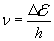## 6. De Broglie hypothesis.

The study of phenomena of absorption and emission of light or, more generally, of electromagnetic waves energy by perfectly absorbent surface (a black body) led M. Planck to the conclusion that these phenomena cannot be explained by continuous processes like those described by (3.9) e (3.10). To avoid theoretical and experimental paradoxes we have to admit that the energy is absorbed and emitted only by multiples of an elementary unit Δ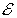proportional to the frequency ν (greek 'nu') of the wave: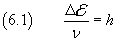The proportionality constant h, by the name of its discoverer called Planck's constant, according to the most recent and accurate measure has value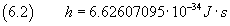So, emission and absorption of energy, even when the propagation of energy is due to waves, occur in a manner typical of the particles, that is with exchange of discrete quantities, since then said energy quanta.

Such a conclusion was inconceivable to contemporary physicists and to Planck himself, convinced of mutual incompatibility between corpuscular and ondulatory processes. It seemed only a provisional expedient until a more satisfactory theory could be established.

But the study of other phenomena, like the photoelectric effect or the Compton effect, convinced the majority of physicists that the results of Planck were not only mathematically but also phisically correct and that was the idea of the incompatibility between corpuscular and ondulatory processes to be wrong.

Among them L. De Broglie hypothesized that physical objects like, for example, electrons, conceived until then as particles, could have ondulatory properties, like wavelength, and ondulatory bahavior, like interference.

In particular, De Broglie supposed that every particle with momentum of magnitude p has wavelength λ inversely proportional to it: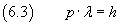Such hypothesis, as well as being consistent with the model of hydrogen atom proposed by N. Bohr to explain the series of rows in its spectrum, was very early supported by experimental controls performed by C. Davisson and L. Germer which showed that flows of electrons can produce interference figures from which evaluate their wavelength.

Then a problem arose: if a particle has ondulatory properties like the wavelength, what is its wave function?

To deal with this problem in a quite simple situation, let us consider a particle with mass m forced to move in space bounded by two hard parallel walls. Let the particle have speed v perpendicular to the walls.

bounching
 Your browser doesn't implement the `canvas` tag.

The classical not relativistic description (which coincides with the intuitive one) of the particle motion is that the particle moves along a straight line with constant speed and that the speed direction changes whenever the mass hits the walls.

Let L be the distance of the walls and T the time interval between two successive hits on the same wall. If at the beginning the particle is in contact with the left wall and has speed directed toward the right wall, the motion of the particle may be represented by a graph such the following: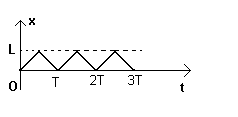The graph shows that we can know the position (the x) and the velocity (the slope) at any instant (except when the mass hits the walls because in this last case there isn't slope, but this is an ideal motion).

Moreover, if there isn't potential energy, classical mechanics states that the total energy of the particle coincides with the kinetic energy: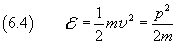This energy, in principle, can take any value from 0 to infinity.

But if we assume the De Broglie hypothesis, we have to think the particle as a standing wave, like those on strings of musical instruments, described by equations like (5.11).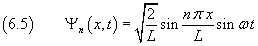A wave is a phenomenon that covers the whole medium: we can know its speed, but not define a point where it is. We know only that it is between 0 and L.

Moreover, if we apply (6.3) in (6.4), we have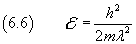The wavelength of a standing wave is given by (5.1), so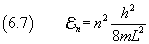This result shows that if a particle behaves like a wave, its energy cannot continuously vary from 0 to infinity, but can have only discrete values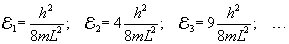In particular the energy cannot be null: its minimum value is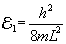If m and L are big with respect to h, the values of the energy are very close each other. When we study macroscopic bodies, masses and displacements are so big with respect to h and consequently energy variations so small, that we can think that the energy varies in a continuous way. But when we study microscopic bodies, like electrons, confined in a very small space like atomic volume, this is no longer true.

These findings agree with those obtained by Planck's analysis of black body radiation and with those obtained by Bohr for the spectrum of hydrogen atom.

If, for example, a particle with energy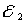jumps to the energy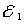, it loses a discrete quantity of energy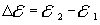. The lost energy propagates as electromagnetic wave with frequency